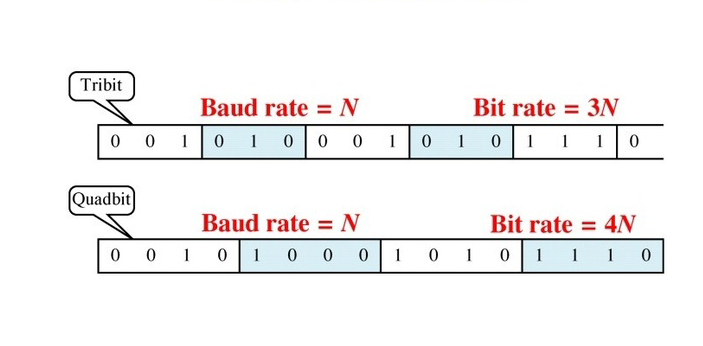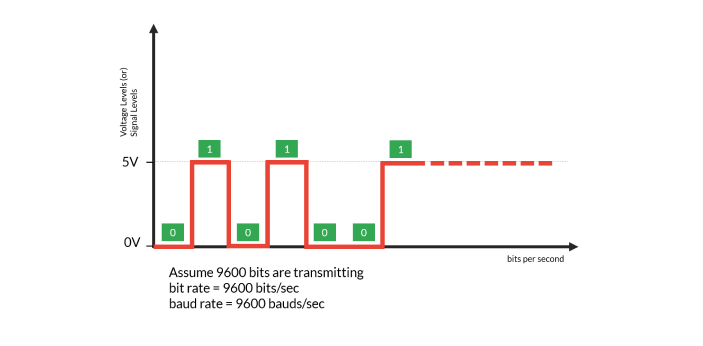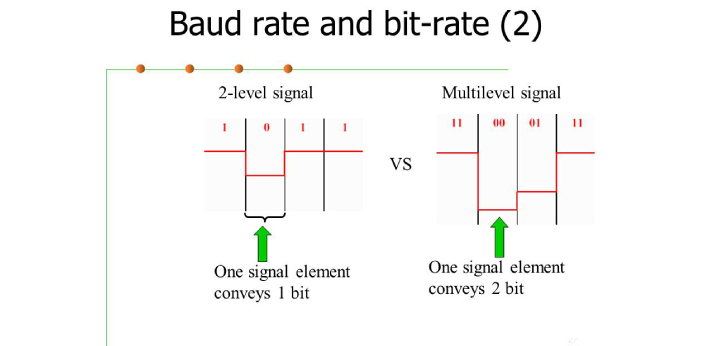What is the difference between Baud Rate and Bitrate? - ProProfs DiscussTopicsMoreProductsMore+ Ask Question

# What is the difference between Baud Rate and Bitrate?

Asked by S. Barnes, Last updated: May 30, 2020

###Request 0FollowShareAnswer AnonymouslyAnswer LaterCopy Link#### N. Reyes

A good writer and orator as well.

N. Reyes, Writer, B.ed, CaliforniaBit rate and baud rate are two types of rates that are important to data communication. Bit rate is the amount of numbers that are transmitted per second, while baud rates are the amount of signal units per second. To determine them, they both use different formulas.The formula for the bid rate is baud rate x the number of bit per baud. The formula for baud rate is bit rate / the number of bit per baud. Another difference between them is how each rate is used. Bid rates are used to test the efficiency of a computer, while the baud rate is used to test the transmission of data.#### J. Lautner

J. Lautner, Product Manager, Utah

Bit rate and baud rate are highly different from each other. The bit rate refers to the number of bits that are transmitted for every second that the internet is being used. The higher the bit rate, the faster the transfer of the files from one gadget to another.The baud rate refers to the signal units that are transferred for every second. Based on these details, you know that the bit rate is faster as compared to the baud rate. There are instances when the baud rate is equal to the bit rate but it will never become greater than the bit rate. Bit rate and baud rate can be expressed using different equations.#### R. Barnes

Always excited to learn and talk about new topics

R. Barnes, Analyst, PhD, Clinton

There are some differences between baud rate and bitrate. Anytime you see the word 'rate' forming a single term with another word, you should know that the term is used in measuring something. Baud rate is used to know the total number of signal units or symbols transmitted in a second. While bitrate is used when you want to know the total number of transmitted bits per second. Bitrate is usually higher than or equal to baud rate, simply because, a single unit being transmitted in a second contains one or more bits.This shows that, the value of bitrate will be higher than that of baud rate. The value of baud rate is limited by the total number of bandwidth given to it. Bitrate, on the other hand, is also limited by the total number of bandwidth allocated to the baud rate, but since a single unit contains one or more bits, the bandwidth for bitrate will also be higher than that of baud rate.Search for Google imagesSelect a recommended image
Upload from your computerCancelSearch for Google imagesSelect a recommended image
Upload from your computerCancelSearch for Google imagesSelect a recommended image
Upload from your computerCancel